Basic Brushless motor Basic Brushless motor | Delta Line# Basic Brushless motor

Basic Technical Knowledge / Basic Brushless motorIf current is caused to flow in the armature conductors, torque is produced.There is an application of a law of physics which is expressed as: F = KBli Where: F = force - K = a constant - B = air gap flux density - l = length of the conductor - i = current in a conductor If more than one conductor is carrying the same current (multiple turns per coil), than F = KBliz Where Z = number of conductors is series.In a motor the conductors rotate abput a central shaft (see figure 1). Than torque, T = FR, where R = radius at the air gap. So, T = KRBliz Figure 1 shows the coil in the zero torque position. The maximum torque position is 90 electrical degrees from the position shown. As the conductors ratate from the maximum torque position, torque drops off in a sinusoidal fashion and becomes zero when the coil has moved 90 degrees.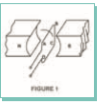A brush type motor has more than one coil. Each coil is angularly displaced from one another so that when the torque from one coil has dropped off, current is automatically switched to another coil wich is properly located to produce maximum torque. The switching is accomplished mechanically with brushes and a commutator as shown in Figure 2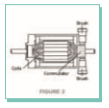In a brushless motor, the position of the coils (phases), with respect to the  permanent magnet field, is sensed electronically and the current is switched, or commutated, to the appropriate pahases. The commutation is effected by means of transistor switches. A brush type motor may be converted into a brushless motor by bringing out all the leads that are attached to the mechanical commutator and providing switches for each lead; however, this approach would involve a large number of switches. Instead, a polyphase winding similar to that used in AC motors is utilized. In this design, the phases are commutated as a function of shaft position.Two, three and four phase motor design are common. This configuration optimizes performance even though it requires more electronic components. Three types of three phase windings are available: Delta bipolar, wye unipolar and wye bipolar. These three winding configurations and their transistor orientation are shown in Figure 3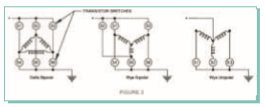Figure 4 illustrates the sequential steps in the commutation of a three phase,  bi-polar system. Closing the transistors 1 and 4 will enable current to flow through phase A and B. The permanent magnet rotor will then align itself in a zero  torque,preferred position. If 1 is opened and 5 closed, current will flow through phases B and C, the rotor will move 120 electrical degrees. (Note that the current through phase A is now flowing in the direction opposite the one at the start of this example.) Obviously, there must be some logic in the order and rate the transistor are switched. Hall Effect sensors are typically used in the logic scheme.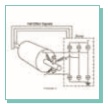Graph 1 may help illustrate how this works.5. For istance, if one were to energize individual phases of a three phase brushless motor one would generate, as a function of electrical degrees of rotation, a torque curve as shown in Graph 1. Each phase would be 120 electrical degrees apart. (It should be noted that elelctrical degrees is simply mechanical degrees  multiplied by the number of pole pairs of the motor). Now, imagine the rotor in Figure 4 resting in its zero torque position (i.e. the 180 electrical degree point of the Graph 1), with current flowing trough winding A. If the rotor is physically moved back from its rest position, torque will build up roughly sinusoidally and become peak at 90 electrical degrees. Since the objective is to have the motor run at its peak operating point, the position still another 30 degrees back from the peack torque point, or 60 degrees, is the point at which the winding must be switched on.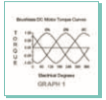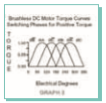A sensor is located to trigger from a rotor magnet at this specific event. If the roor is allowed to turn back towards its original rest, or zero torque point, but the current is switched from winding A to winding B at 180 electrical degrees, the motor will operate on a new sine wave, or torque vs. angle, resulting in another point of peak performance. Again, a sensor is located in such a manner to mark this event. Similarly, the third sensor is set to trigger at 300 electrical degrees. These Hall Effect sensor setting, 120 electrical degrees apart from sensor to  sensor, automatically sequence the switching of currents from one phase to another, at the appropriate time. Another important point to note from Graph 1 is the sign of the torque generated as a function of rotor position. If the currents in  individual phases were switched at the proper electrical position, positive torque could always be generated, as illustrated in Graph 2. With the proper selection of phase energization (i.e. the proper commutation scheme) the resultant torque output of the motor is as illustrated in Graph 3. The successful commutation of the brushless motor is knowing the rotor position in electrical degrees and having the proper commutation scheme.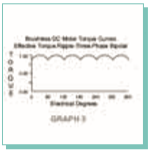The nature of the application under consideration dictates what information is  required to properly select a motor candidate. For example, operating at a fixed speed will have a different demand than  operation under servo conditions. In general, three parameters will determine motor selection: 1 Peak torque requirement 2 RMS torque requirement 3 Speed of operationPEAK TORQUE REQUIREMENT Peak torque Tp is the sum of the torque due to acceleration of inertia, Tj, load, Ti, and friction Tf: Tp=Tj+Ti+Tf Looking at the separate components, the torque due to inertia is the product of the load (including motor rotor) inertia and the load acceleration: Tj=J*a (a = acceleration) The torque due to the load is defined by the configuration of the mechanical system coupled to the motor. The system also determines the amount of torque required to overcome the friction.RMS TORQUE REQUIREMENT Root-Mean-Square or RMS torque is a value used to approximate the average continuous torque requirement. It is a statistical approximation described by the following equation Where t1 is the acceleration time, t2 is the run time, t3 is the deceleration time, and t4 is the time in a move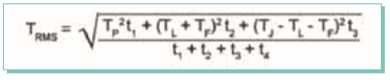SPEED OF OPERATION Speed of operation is also dictated bythe configuration of the mechanical system that is coupled to the motor shaft, and by the type of move that is to be effected. For example, a single speed application would require a motor with rated speed equal to the average move speed. A point to point positioning application would require a motor with a rated speed higher than the average move speed. (The higher speed would account for acceleration , deceleration and run times of the motion profile). Figure 8A and 8B relate rated operating speed to average move speed for point to point positioning move profiles.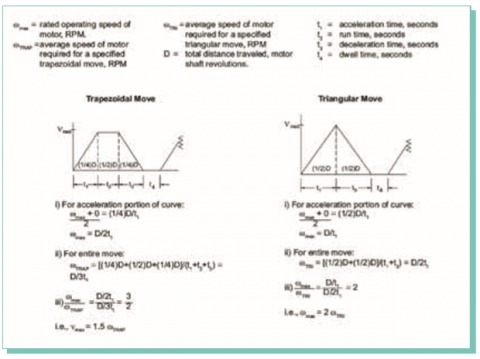Switzerland - Global HQ

Via Prè d’là, 1
CH-6814 Lamone, Switzerland
Phone: +41 (0)91 612 85 00
Fax: +41 (0)91 612 85 19
E-mail: info@delta-line.com
USA

Delta Line North America, Inc
DTC Tech
4600 South Syracuse, 9th Floor
Denver, CO 80237, USA
Phone: +1 303 256 6212
E-mail: infous@delta-line.com
China

Company Limited,
414 Building 1,
FuChen HuaYuan
New North District Changzhou,
Jiangsu, China
© 2020 All rights reserved|Privacy & cookie Policy
Powered by Ico Computer|Sitemap